# Test: Electrical Circuits- 3 (Hindi)

## 20 Questions MCQ Test Mock Test Series for SSC JE Electrical Engineering (Hindi) | Test: Electrical Circuits- 3 (Hindi)

Description
Attempt Test: Electrical Circuits- 3 (Hindi) | 20 questions in 12 minutes | Mock test for SSC preparation | Free important questions MCQ to study Mock Test Series for SSC JE Electrical Engineering (Hindi) for SSC Exam | Download free PDF with solutions
QUESTION: 1

### If the length of a wire having uniform resistance R is stretched in m times with volume remaining same. What will be new resistance value?

Solution:

Given that, the length of a wire having uniform resistance R is stretched in m times with volume remaining same.

Let the length of the wire is L1

and the area of the wire is A1

For volume remains to be same

L1A1 = L2A2

⇒ L11 = (mL1) (A2)

⇒ A1 = mA2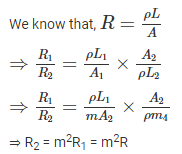QUESTION: 2

### A filament lamp of 40 W, 110 V is connected in series with resistance R. if the supply voltage is 230 V, the value of resistance R should be:

Solution:

Given that, P = 40 W, V = 110 V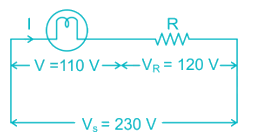Voltage across resistor = Vs - V = 230 - 110 = 120 V

Power rating of lamp = 40

⇒ VI = 40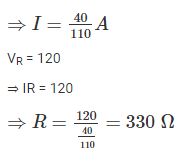QUESTION: 3

### The temperature coefficient of resistance of material is given as:

Solution:

We know that, R = R0[1+α(T1−T2)]

Where α is a temperature coefficient of resistance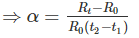If the temperature coefficient of resistance is positive, it indicates that the resistance increases with the increase in temperature

If the temperature coefficient of resistance is negative, it indicates that the resistance decreases with the increase in temperature

QUESTION: 4

Which of the following is not the same as watt?

Solution:

Watt is the unit of power. We can represent the power as follows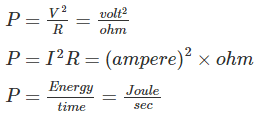Hence Volt/Ampere is not same as watt, it is equal to ohm.

QUESTION: 5

Find the average current flow in the wire.

Given: type of wire: metallic

Total electrons drift across section per second = 1020

Solution:

Total electrons drift across section power second = 1020

We know that, q = it

For t = 1 sec

⇒ q = i

⇒ i = q = ne = 1020 × 1.6 × 10-19 = 16 A

QUESTION: 6

Three equal resistors of 50 Ω each are connected in parallel across 110 V dc supply. What is the current through each branch if one of the resistors gets shorted?

Solution: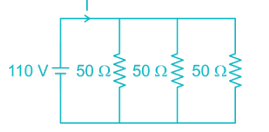If one of the resistors gest shorted, remaining two resistors also gets shorted as they are connected in parallel. Hence the current flow through each branch will be zero.

QUESTION: 7

For a network Thevenin equivalent is given by Vth = 10 V and Rth = 50 Ω. If this network is shunted by another 50 Ω ……… at load. What is the new Thevenin equivalent of the network?

Solution: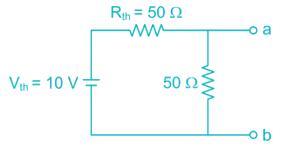Now voltage across terminal a - b is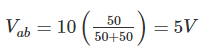The Thevenin’s resistance can be find by neglecting the voltages source.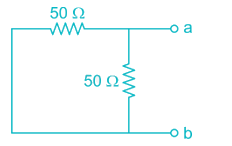Rab = 25 Ω

New Thevenin equivalent network is,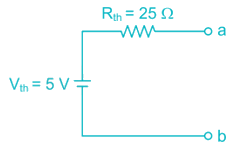QUESTION: 8

A circuit consists of three elements connected in series; 10 V battery, 100 Ω and load resistor RL. The maximum power that can be delivered to load would be?

Solution: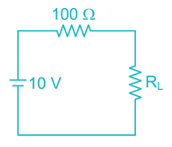For maximum power transfer, RL = Rth = 100 Ω

Maximumpower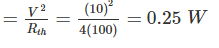QUESTION: 9

Which of the following statements is/are correct about Norton Theorem?

A) The value of the current source is the short circuit current between the two terminals of the network.

B) Resistance is the equivalent resistance measured between the terminals of the network with all the energy. Sources are replaced by their internal resistance.

Solution:

Norton’s network for any given network can be represented as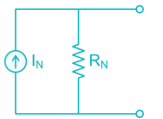The value of the current source is the short circuit current between the two terminals of the network

Norton’s Resistance is the equivalent resistance measured between the terminals of the network with all the energy. Sources are replaced by their internal resistance.

QUESTION: 10

A current source and a voltage source are connected in parallel with a resistor as shown below. Suppose that vs = 15 V, is = 5A and R = 5 Ω. What is the current i in the resistor and the power absorbed by the resistor?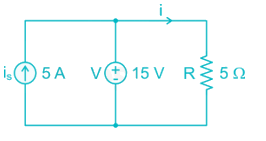Solution:

Voltage across resistor R = 15 V

Current(I) = 15/5 = 3A

Power absorbed by resistor =(15)2/5 = 45W

QUESTION: 11

For the network shown in the following figure the value of Vs which makes I0 = 7.5 mA is: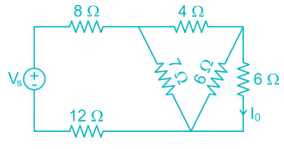Solution: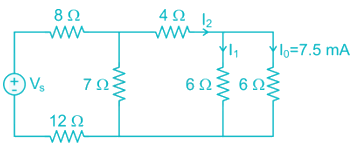Given that, I0 = 7.5 mA

Hence, I1 = 7.5 mA

I2 = I0 + I1 = 15 mA

Now we can reduce the diagram,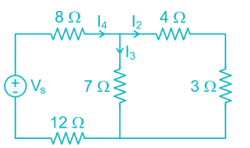As, I2 = 15 mA, I3 = 15 mA

I4 = I2 + I3 = 30 mA

Now, the circuit becomes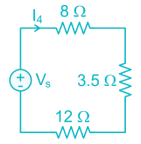⇒ Vs = I4 (Req) = 30 × 10-3 × (8 + 3.5 + 12) = 705 mV

QUESTION: 12

Which statement about electrical network and electrical circuit is CORRECT?

Solution:

An Electrical network is an interconnection of various electrical elements.

An electrical circuit is a network which has a closed path that gives the return path for the flow of current.

Electrical networks can either be closed or open.

Hence every electrical circuit is a network, but all networks are not circuits.

QUESTION: 13

In the figure given below, the initial capacitor voltage is zero. Then after switch is closes at t = 0, Calculate the final steady state voltage across the circuit: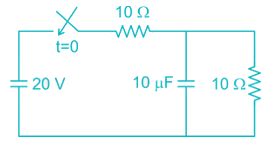Solution:

At steady state, the capacitor acts as open circuit.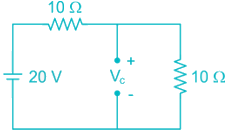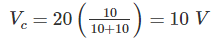QUESTION: 14

Consider the following given figure and calculate steady-state value of v(t) if i(t) is a unit step current.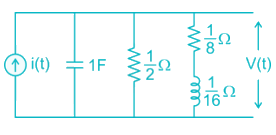Solution:

At steady state inductor acts as short circuit and the capacitor acts as open circuit.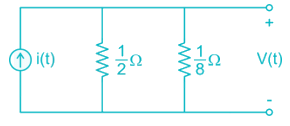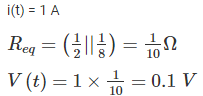QUESTION: 15

An ac voltage has frequency of 50 Hz with peak amplitude of 100 V. In how many seconds after the zero value, voltage will attain the value of 86.6 V?

Solution:

Given that, Vm = 100 V

V = 86.6 V

f = 50 ⇒ ω = 2πf = 100 π

V = Vm sin ωt

⇒ 86.6 = 100 sin (100 πt)

⇒ sin (100 πt) = 0.866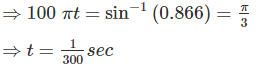QUESTION: 16

In an RL series circuit, the current flowing is 5 A, the voltage drop across R is 16 V and the voltage drop across L is 12 V. Then what is the impedance?

Solution:

Given that, I = 5A, VR = 16 V, VL = 12 V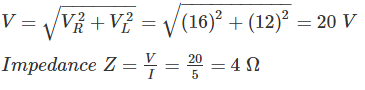QUESTION: 17

Express the voltage shown below in the general form v(t) = Vm sin (ωt + θ).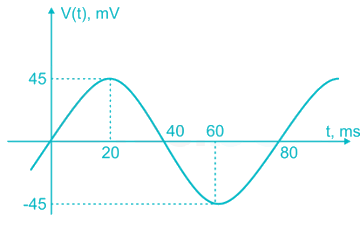Solution:

V(t) = Vm sin (ωt + θ)

From the given wave form,

Vm = 45

Time period, T = 80 ms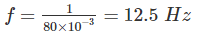ω = 2πf = 25 π

V(t) = 45 sin (25 πt + 0)

QUESTION: 18

A current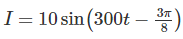is produced when a voltage V = 30 sin 300 t is applied to a circuit. The estimated value of impedance is:

Solution: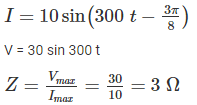QUESTION: 19

Two circuits having the same magnitudes of impedances are joined in parallel. The power factor of one circuit is 0.8 and of other is 0.6. The power factor of the combination is -

Solution:

Power factor of first circuit = 0.8 (cos ϕ1)

Power factor of second circuit = 0.6 (cos ϕ2)

The power factor of parallel combination,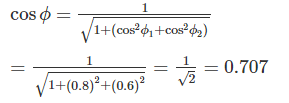QUESTION: 20

For parallel RLC circuit, which of the following statements is not correct?

Solution:

1) The bandwidth of a parallel RLC circuit is decreases by increasing the resistance

2) The bandwidth is independent of inductance. Hence the bandwidth of the circuit remains the same, if L is increased

3) At resonance the impedance is equal to resistance, it behaves as a pure resistive circuit. Hence the input impedance is a real quantity

4) At resonance, the impedance is equal to resistance. It is the maximum value of impedance and hence current attains its minimumUse Code STAYHOME200 and get INR 200 additional OFF Use Coupon Code